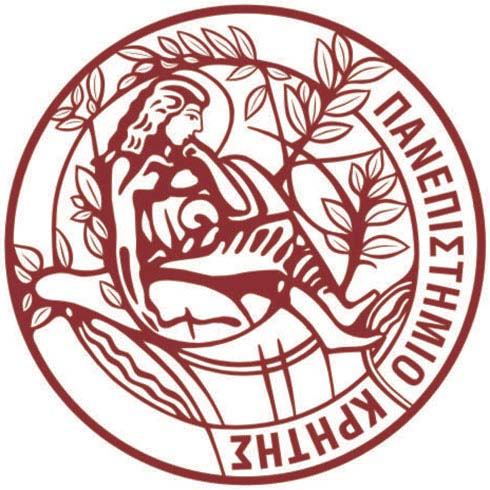University of CreteDepartment of Mathematics and Applied Mathematics

Panagiotis Chatzipantelidis

Associate Professor

Research

Research Interests
• Numerical solution of PDE's: Finite Element Methods, Finite Volume Methods
• Numerical solution of stochastic partial differential equations

Publications

•  Books
1. Numerical Solution of Partial Differential Equations, (with M. Plexousakis), (pdf, html), (electronic book in greek, location:
http://hdl.handle.net/11419/665), Hellenic Academic Ebooks, Athens, (2016).
• Research papers
1. Explicit multistep methods for nonstiff partial differential equations, Applied Numerical Mathematics, 27, (1998), 13-31.
2. A finite volume method based on the Crouzeix-Raviart element for  elliptic pde's in two dimensions, Numeriche Mathematik, 82, (1999), 409-432.
3. Finite volume methods for elliptic pde's: A new approach, M2AN Mathematical Modelling and Numerical Analysis, 36, (2002), 307-324.
4. On solving elliptic stochastic partial differential equations, (with I. Babuska), Computer Methods in Applied Mechanics and  Engineering, 191, (2002), 4093-4122.
5. The finite volume element method in nonconvex polygonal domains, (with R. Lazarov), Finite Volumes for Complex Applications III, ed. R. Herbin and D. Kroner, Hermes Penton Science, London, (2002), 171--178. (Refereed conference).
6. A-posteriori error estimates for a finite volume method for the Stokes problem in two dimensions, (with Ch. Makridakis and M. Plexousakis), Applied Numerical Mathematics46, (2003), 45-58.
7. Error estimates for a finite volume element method for parabolic equations in convex polygonal domains, (with R. Lazarov and V. Thomee), Numerical Methods for PDE's, 20, (2004), 650-674, and Technical Report ISC-MATH-03-03, Texas A&M University.
8. Error estimates for a  finite volume element method for elliptic pde's in nonconvex polygonal domains, (with R. Lazarov),  SIAM Journal on Numerical Analysis, 42, (2005), 1932-1958, and Technical Report ISC-MATH-03-04, Texas A&M University.
9. A finite volume element method for a nonlinear elliptic problem, (with R. Lazarov and V. Ginting), Numerical Linear Algebra with Applications, 12, 515-546, (2005), and  Technical Report ISC-MATH-04-06, Texas A&M University.
10. Parabolic finite element equations in nonconvex polygonal domains, (with R. LazarovV. Thomee and L.B. Wahlbin), BIT  Numerical Mathematics, 46, 113-143, (2006).
11. Parabolic finite volume element equations in nonconvex  polygonal domains, (with R. Lazarov and  V. Thomee ), Numerical Methods for PDE's, 25, 507-525, (2009).
12. SIAM Journal on Numerical Analysis, 48 (2010), 109-132.
13. Some error estimates for the lumped mass finite element method for a parabolic problem, (with R. Lazarov and  V. Thomee),  Mathematics of Computation81, 1-20, (2012).
14. Some error estimates for the  finite volume element method for a parabolic problem, (with R. Lazarov and  V. Thomee),  Computational Methods in Applied Mathematics, 13, 251-279, (2013).
15. A finite volume element method for a nonlinear parabolic problem, (with V. Ginting),  Numerical Solution of Partial Differential Equations: Theory, Algorithms, and Their Applications, Springer Proceedings in Mathematics & Statistics, Vol. 45,  121–136, (2013).
16. On preservation of positivity in some finite element methods for the heat equation, (with Z. Horvarth and  V. Thomee),  Computational Methods in Applied Mathematics, 15, 417-437, (2015).